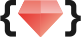# 五倍紅寶石 ・專業程式教育機構## 撰寫正規表達式

``````// 1. 使用 literal，這種方式會在 script 載入時就被編譯，效能較好。
const regex = /some text/

// 2. 使用 new 建構一個 RegExp 物件，適合用在需要動態產生 pattern 的場合。
const regex = new RegExp('some text')

// 加上 flag 設定，使比對的能力更強大。i：不區分大小寫，g：比對字串所有位置
const regex = /some text/i
const regex = new RegExp('some text', 'g')``````

## 使用正規表達式

``````const regex = /hello world/i

// 使用 test 比對字串是否符合 pattern，回傳 boolean
regex.test('Hello World !!') // true

// 使用 exec 取得比對的詳細資訊，比對失敗時回傳 null
regex.exec('Hello World !!') // ["Hello World", index: 0, input: "Hello World !!", groups: undefined]
regex.exec('Hello Regex !!') // null``````

`String 物件` 中的 `search``match``replace``split` 等方法中，也有支援正規表達式寫法。

``````const paragraph = 'Lorem Ipsum is simply dummy text of the printing and typesetting industry.'

// 使用 search 搜尋字串是否在段落中，有找到回傳字串的起始位置，沒找到回傳 -1
paragraph.search('tExT') // -1
paragraph.search(/tExT/i) // 28

// 使用 match 找出第一個比對成功的詳細資訊，加上 g flag 則會列出所有比對成功的字串
paragraph.match(/ing/) // ["ing", index: 45, ...]
paragraph.match(/ing/g) // ["ing", "ing"]``````

## 特殊字元

``````const  str ='Rails is a web framework written in Ruby'

// ^ 表示 pattern 必須在字串的開頭
str.match(/^Rails/) // ["Rails", index: 0, ...]
str.match(/^Ruby/) // null

// \$ 表示 pattern 必須在字串的結尾
str.match(/Ruby\$/) // ["Ruby", index: 36, ...]
str.match(/Rails\$/) // null

// | 表示 或(or)， | 前後的字串都可以比對
const regex = /color|colour/
regex.exec('color') // ["color", index: 0, ...]
regex.exec('colour') // ["colour", index: 0, ...]

// 當要比對這些特殊符號時，使用反斜線'\'來跳脫特殊字元
const regex = /\\$100/
regex.test('\$100') // true``````

## 集合 `[]`

``````// 只要是英文大寫字母，就比對成功
const regex = /[ABCDEFGHIJKLMNOPQRSTUVWXYZ]/
'K'.match(regex) // ["K", index: 0, ...]
'δ'.match(regex) // null

// 可以使用 '-' 來簡化集合，'A-Z' 表示英文字母 A ~ Z 都符合
const regex = /[A-Z]/

// 若要比對的是英文或數字，可以這樣表示
const regex = /[A-Za-z0-9]/``````

``````const regex = /./   // 比對換行符號外的任意一個字元
const regex = /\d/  // 比對一個數字，相等於 /[0-9]/
const regex = /\w/  // 比對一個英文、數字或底線，相等於 /[A-Za-z0-9_]/
const regex = /\s/  // 比對一個的空格 (ex: space, tab, 換行, ...)``````

``````const regex = /[^\w]/
regex.test('a') // false
regex.test('!') // true``````

## 量詞 `{}`

``````// 不使用量詞時，要比對 5 個連續的數字就必須寫 5 次
const regex = /\d\d\d\d\d/
regex.test('12345') // true

// 使用 {5} 表示連續出現 5 次
const regex = /\d{5}/
regex.exec('abcde12345') // ["12345", index: 5, ...]
regex.exec('a1b2c3d4e5') // null

// 使用 {2,} 表示連續出現 2 次以上
const regex = /\w\+{2,}/
regex.exec('a+') // null
regex.exec('a++') // ["a++", index: 0, ...]

// 使用 {2, 5} 表示連續出現 2 ~ 5 次
const regex = /^\w{2,5}!/
regex.exec('Hi!') // ["Hi!", index: 0, ...]
regex.exec('Helloooo!') // null``````

``````// 使用 ? 表示出現 0 或 1 次，等同於 {0,1}
const regex = /\w?/
// 使用 + 表示出現 1 次或以上，等同於 {1,}
const regex = /\w+/
// 使用 * 表示出現 0 次或以上，等同於 {0,}
const regex = /\w*/``````

``````// '+' 出現的次數越多優先
const regex = /a\+{2,}/
regex.exec('a+++++') // ["a+++++", index: 0, ...]
// '+' 出現的次數越少優先
const regex = /a\+{2,}?/
regex.exec('a+++++') // ["a++", index: 0, ...]``````

## 練習1

### 1 使用正規表達式來檢驗日期格式是否正確。

``````// 假設正確的日期格式為 '2020/07/15'
// 分析一下是由 "4個數字/2個數字/2個數字" 所組成，
// 把這個規則寫成正規表達式
const regex = /\d{4}\/\d{2}\/\d{2}/

// 若要支援'2020/07/15'、'2020-07-15'兩種寫法，可以使用集合來處理
const regex = /\d{4}[/\-]\d{2}[/\-]\d{2}/
regex.test('2020/07/15') // true
regex.test('2020-07-15') // true``````

### 2 使用正規表達式解析 excel 複製來的資料。

``````// 假設使用者在 excel 選取了 'sn0001'、'sn0002'、'sn0003' 三個欄位
// 分析一下我們要的每一筆資料是由 "連續的英文或數字" 所組成
// 把這個規則寫成正規表達式
const regex = /[A-Za-z0-9]+/

// 在複製文字的時候會發現，除了流水號之外，分隔、換行符號也一起被複製了進來
// 用 replace 去 trim 掉不需要的字元在處理上比較麻煩
// 改使用 match 一次取出所有資料，並轉成陣列供後續使用
const copiedData = `
sn001,
sn002,
sn003
`
copiedData.match(/[A-Za-z0-9]+/g) // ["sn001", "sn002", "sn003"]``````

### 3 使用正規表達式找出被 `* *` 包起來的片段。

``````const paragraph= "Lorem Ipsum is simply dummy text of the *printing* and *typesetting* industry."
// 分析一下我們要的資訊是由 '*' + 任意內容 + '*' 所組成
// 把這個規則寫成正規表達式
const regex = /\*.*\*/g
paragraph.match(regex) // ["*printing* and *typesetting*"]

// 執行之後會發現結果不如預期
// 原因在於中間的任意內容我們是使用 Greedy量詞 '*' 去比對，也就是任意字元且越多越好
// 改使用 Lazy 量詞就能獲得想要的結果
const regex = /\*.*?\*/g
paragraph.match(regex) // ["*printing*", "*typesetting*"]``````

## 群組 (Capturing Group)

``````// 在使用 exec、match 等方法時，回傳的結果會有以下這些資訊
// [比對成功的字串, 捕獲的字串1, 捕獲的字串2, ..., 字串的起始位置, 命名的捕獲群組]
const regex = /user: (\w+)/
regex.exec('user: Alan') // ["user: Alan", "Alan", index: 0, ...]

// 每一個 Group 會 '由左至右，由外而內' 的被賦予編號，並被放在執行結果相應的 index 中
// 假設 regex = /((A)(B))/ ，那麼群組就分別是 1:(AB), 2:(A), 3:(B)
const regex = /fullName: ((\w+) (\w+))/
regex.exec('fullName: Alan Hsu') // ["fullName: Alan Hsu", "Alan Hsu", "Alan", "Hsu", ...]``````

``````// 假設想找出猜數字中 a 和 b 出現次數相同的回合
// 作法1 → 我們可以先把 a 與 b 的次數 Group 起來，再做判斷
const regex = /(\d+)a(\d+)b/
const result = regex.exec('1a1b') // ["1a1b", "1", "1", ...]
if (result === result) ...

// 作法2 → 使用 backreference，讓 \1、\2、... 替換成被捕獲的數字
const regex = /(\d+)a\1b/
regex.test('1a1b') // true
regex.test('1a2b') // false``````

``````// 假設要抓出使用者的 firstName 與 lastName
const regex = /fullName: (?<firstName>\w+) (?<lastName>\w+)/
regex.exec('fullName: Alan Hsu') // [...,  groups: {firstName: "Alan", lastName: "Hsu"}]``````

``````// 使用群組簡化 | 的寫法
const regex = /good apple|bad apple/

// 使用量詞簡化重複的規則
const regex = /\d{1,3}\.\d{1,3}\.\d{1,3}\.\d{1,3}/
const regex = /(\d{1,3}\.){3}\d{1,3}/
regex.exec('192.168.0.1') // ["192.168.0.1", "0.", index: 0, ...]``````

``````// 使用 non-capturing group，(?: )內的文字就不會被捕獲，也就無法使用 backreference
const regex = /(?:\d{1,3}\.){3}\d{1,3}/
regex.exec('192.168.0.1') // ["192.168.0.1", index: 0, ...]``````

## 斷言 (Assertions)

``````// 假設要找出 'Java' 而不是 'Javascript'
const str = 'difference between Javascript and Java.'
// 不使用斷言會找到 'Javascript' 的 'Java'
str.match(/Java/) // ["Java", index: 19, ...]
// 改使用文字邊界 \b 來比對，就能找到想要的結果了
str.match(/\bJava\b/) // ["Java", index: 34, ...]

// 文字邊界指的是在比對到 \b 的位置時，前後相鄰的字元必須有一個不是文字
// 使用 replace 把 \b 替換成 '|' 來看看它的效果
const regex = /\b/g
str.replace(regex, '|') // "|difference| |between| |Javascript| |and| |Java|."``````

``````// Lookaround 分為兩種 `Lookahead` 以及 `Lookbehind`，各自又有 positive 與 negative 兩種判斷方式
// Positive Lookahead： A(?=B) → A 後方的條件要符合 B
// Negative Lookahead： A(?!B) → A 後方的條件不能符合 B
// Positive Lookbehind： (?<=A)B → B 前方的條件要符合 A
// Negative Lookbehind： (?<!A)B → B 前方的條件不能符合 A

// 假設要取出商品的金額
const str = '數量 2,實付金額 990元'
// 分析一下要擷取的資料 "前方有一個空格 + 金額 + 後方有一個'元'"
// 把規則寫成正規表達式
const regex = /(?<=\s)\d+(?=元)/
str.match(regex) // ["990", index: 10, ...]``````

## 練習2

### 1 使用正規表達式來轉換日期。

``````// 將日期 '2020/07/15' 轉換為 '2020年07月15日'
const date = '2020/07/15'
// 使用 group 把年、月、日抽取出來
const regex = /(\d{4})\/(\d{2})\/(\d{2})/
// 使用 replace + backreference 轉換字串
// 在 replace 中是使用 \$1、\$2、... 替代群組捕獲的文字
date.replace(regex,'\$1年\$2月\$3日') // "2020年07月15日"

// 使用命名群組讓程式碼更好讀
// 在 replace 中是使用 \$<name> 替代命名群組捕獲的文字
const regex = /(?<year>\d{4})\/(?<month>\d{2})\/(?<day>\d{2})/
date.replace(regex,'\$<year>年\$<month>月\$<day>日') // "2020年07月15日"``````

### 2 使用正規表達式幫數字加上三位一撇 (separator)。

``````const number = 123456789 // 123,456,789
// 分析一下條件，從個位數開始數，每三位數加上一個','
// 使用非文字邊界 \B 與 Lookahead 來撰寫條件，判斷右邊每 3 個數字為錨點
const regex = /\B(?=(?:\d{3})+)/g
number.toString().replace(regex, ',') // "1,2,3,4,5,6,789"

// 發現結果不如預期，從數字 5 開始往右數三個數字也符合條件
// 判斷每湊滿 3、6、9、... 個數字之後，右邊不能再有其他數字
const regex = /\B(?=(?:\d{3})+(?!\d))/g
number.toString().replace(regex, ',') // "123,456,789"``````

### 3 使用正規表達式限制使用者只能輸入英文與數字

``````// 分析一下條件，每個字元都只能是英文與數字
// 反過來說就是要 trim 掉不是英文與數字的字元
const value = e.target.value
this.value = value.replace(/[^A-Za-z0-9]/g, '')``````

## 參考資料

👩‍🏫 課務小幫手：

✨ 想掌握 JavaScript 觀念和原理嗎？